8. THE D- AND f-BLOCK ELEMENTS

1. Oxidation number of gold metal is

(A) + 1
(B) O
(C) – 1
(D) all of these

2. Shape of d-orbital is

(A) spherical
(B) dumb bell
(C) double dumb bell
(D) none of these

3. Electronic configuration of alkaline earth element is

(A) ns2
(B) ns1
(C) np6
(D) nso

4. Outermost configuration 3d64s2k is of

(A) Ca
(B) fe
(C) Mg
(D) Cu

5. Which one of the following is called green vitriol ?

(A) FeSO4.7H2O
(B) CuSO4.5H2O
(C) CaSO4.2H2O
(D) None of these

6. Which block of elements are known as transition elements ?

(A) p-block
(B) s-block
(C) d-block
(D) ƒ-block

7. Most abundant element in earth’s crust is

(A) Si
(B) Al
(C) Zn
(D) Fe

8. Which one of the following is diamagnetic ion ?

(A) Co2+
(B) Ni2+
(C) Cu2+
(D) Zn2+

9. Which of the following is not an element of first transition series ?

(A) Iron
(B) Chromium
(C) Magnesium
(D) Nickel

10. The correct order of E0M2+/M values with negative sign for the four successive elements Cr, Mn, Fe and Co is

(A) Fe > Mn > Cr > Co
(B) Cr > Mn > Fe > Co
(C) Mn > Cr > Fe > Co
(D) Cr > Fe > Mn > Co

11. The number of unpaired electrons in gaseous species of Mn3+, Cl3+ and V3+ respectively are …… and the most stable species is ………..

(A) 4, 3 and 2; V3+
(B) 3, 3 and 2; Cr3+
(C) 4, 3 and 2; Cr3+
(D) 3, 3 and 3; Mn3+

12. The melting points of Cu, Ag and Au follow the order

(A) Cu > Ag > Au
(B) Cu > Au > Ag
(C) Au > Ag > Cu
(D) Ag > Au > Cu

13. In which of the following pairs of ions, the lower oxidation state in aqueous solution is more stable than the other ?

(A) Ti+, Ti3+
(B) Cu+, Cu2+
(C) Cr2+, Cr3+
(D) V2+, VO2+ (V4)

14. Which of the following pairs of ions have the same electronic configuration ?

(A) Cu2+, Cr2+
(B) Fe3+, Mn2+
(c) Co3+, Ni3+
(D) Sc3+, Cr3+

15. In the dichromate anion (Cr2O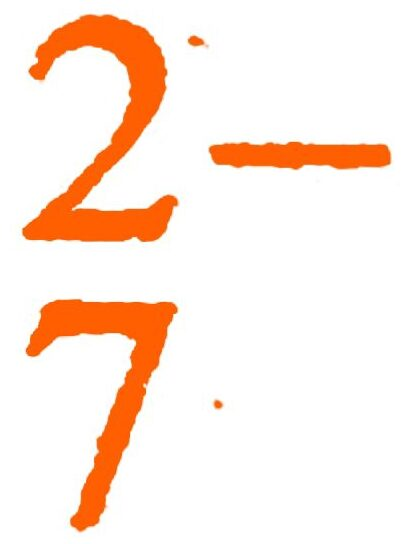)

(A) all Cr – O bonds are equivalent
(B) 6 Cr – O bonds are equivalent
(C) 3 Cr – O bonds are equivalent
(D) no bonds in Cr2O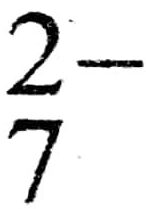are equivalent

16. Which of the following has no unpaired electrons but is coloured ?

(A) K2Cr2O7
(B) K2MnO4
(c) CuSO4 .5H2O
(D) MnCl2

17. V2O5 reacts with akalies as well as acids to give

(A) VO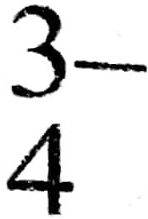and VO2+
(B) VO2+ and Vo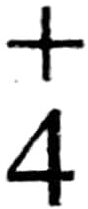(C) VOand VO2+
(D) VOand VO18. Which of the following lanthanide ion is paramagnetic ?

(A) Ce4+
(B) Yb2+
(C) Lu3+
(D) Eu2+

19. Lanthanoid contraction is due to increase in

(A) atomic number
(B) effective nuclear charge
(D) valence electrons

20. The correct configuration of ƒ-block elements are

(A) (n − 2) ƒ1 – 14 (n – 1) do – 1 ns2
(B) (n – 1) ƒ1 – 14 (n – 1) do – 1 ns2
(C) (n – 3) ƒ1 – 14 (n − 2) do – 1 ns2
(D) (n – 2) ƒ० – 1 (n – 1)d do – 1 ns2

21. KMnO4 acts as an oxidising agent in acidic medium. The number of moles of KMnO4 that will be needed to react with one mole of sulphide ions in acidic solution is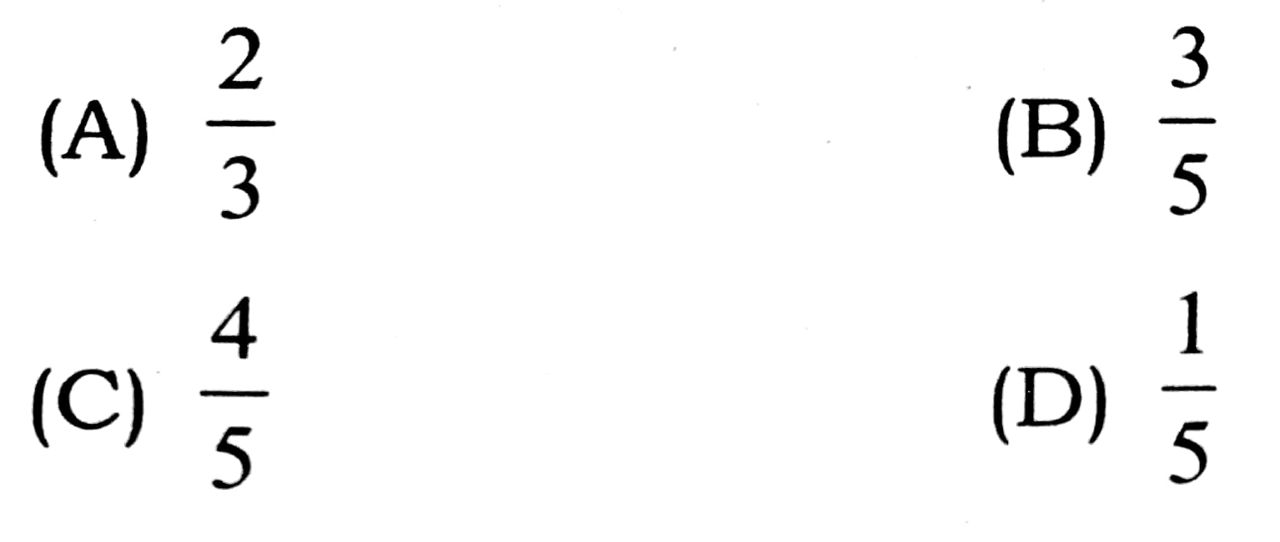22. The general electronic configuration of transition elements are

(A) (n – 1)d5
(B) (n – 1) d(1 – 10)ns0.1 or 2
(C) (n − 1) d(1 – 10)ns0 – 2
(D) none of these

23. The highest magnetic moment shown by the transition metal ion with the outermost electronic configuration is

(A) 3d5
(B) 3d2
(C) 3d7
(D) 3d9

24. Transitor elements are

(A) Metals
(B) Non-metals
(C) a-block elements
(D) Gas

25. Which of the following elements is liquid at room temperature ?

(A) Zn
(B) Hg
(C) Cu
(D) Au

26. Which of the following is known as red lead ?

(A) Pb3O4
(B) Pb2O3
(C) PbO
(D) PbO2